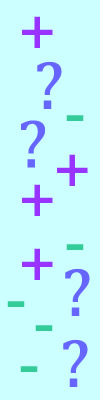#### You may also like### Prompt Cards

These two group activities use mathematical reasoning - one is numerical, one geometric.### Consecutive Numbers

An investigation involving adding and subtracting sets of consecutive numbers. Lots to find out, lots to explore.### Exploring Wild & Wonderful Number Patterns

EWWNP means Exploring Wild and Wonderful Number Patterns Created by Yourself! Investigate what happens if we create number patterns using some simple rules.

# Sums and Differences 2

## Sums and Differences 2This challenge is about adding and subtracting two-digit numbers.

Pick two two-digit numbers.
Find the sum of the numbers.
Find the difference between the numbers.
Then find the sum and difference of the sum and difference.

What do you notice about the numbers?
Does this always happen?
Try for other starting numbers.

For example:
My numbers are 24 and 31.
The sum of them is 55 and the difference between them is 7.
Now my numbers are 55 and 7.
The sum of them is 62 and the difference between them is 48.

#### Why do this activity?

This activity provides a context for practising summing two two-digit numbers, and finding the difference between two two-digit numbers. It encourages children to conjecture about relationships and then test their ideas. Once a pattern is spotted, children should be encouraged to check their answers by using the inverse.

#### Possible approach

It would be good to start the lesson by practising strategies for summing numbers and finding the difference between numbers.

The class could then work through a version of the challenge all together. You could model the following example and invite pairs/groups to try other pairs of numbers:

 1st number 2nd number Sum Difference Sum Difference 24 31 55 7 62 48 14 20 34 6 40 28

As you gather their ideas, you could present them in the table and then invite the class to look along rows and notice any patterns or relationships between the numbers. Is the sum always higher than the difference? Is the sum and difference ever the same? Is there a relationship between the original numbers and the others? Can they predict what will happen for other numbers?

Children may notice a pattern. They should be encouraged to conjecture a rule and go away and check the rule for other pairs of numbers.  Can they explain why this happens?

Some children may move onto the extension task (below).

#### Key questions

What is the sum of the numbers? What is the difference between the numbers?
Can you spot a pattern?
Can you predict what the sum/difference will be? How will you check your calculation?

#### Possible extensions

Extension 1: Add two more columns to your table. If you kept finding the sum and difference, what other patterns do you think might emerge? Look carefully along each row and consider how the new numbers relate to the earlier ones.

 1st number 2nd number Sum Difference Sum Difference Sum Difference 24 31 55 7 62 48 62+48 62-48 14 20 34 6 40 28 40+28 40-28

Extension 2: What if you added another two columns? What if you kept adding columns? How big do you think the numbers will get?

#### Possible support

A 100 square or number line/track would be useful.